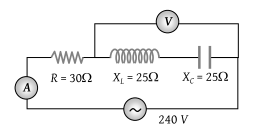# NEET Physics Alternating Current Questions SolvedIn the circuit shown in figure neglecting source resistance the voltmeter and ammeter reading will respectively, will be(1) 0V, 3A

(2) 150V, 3A

(3) 150V, 6A

(4) 0V, 8A

(4) The voltage ${V}_{L}$ and ${V}_{C}$ are equal and opposite so voltmeter reading will be zero.

Also $R=30\text{\hspace{0.17em}}\Omega ,\text{\hspace{0.17em}\hspace{0.17em}}{X}_{L}={X}_{C}=25\Omega$

So $i=\frac{V}{\sqrt{{R}^{2}+{\left({X}_{L}-{X}_{C}\right)}^{2}}}=\frac{V}{R}=\frac{240}{30}=8\text{\hspace{0.17em}}A$

Difficulty Level:

• 5%
• 17%
• 5%
• 75%
Crack NEET with Online Course - Free Trial (Offer Valid Till September 21, 2019)